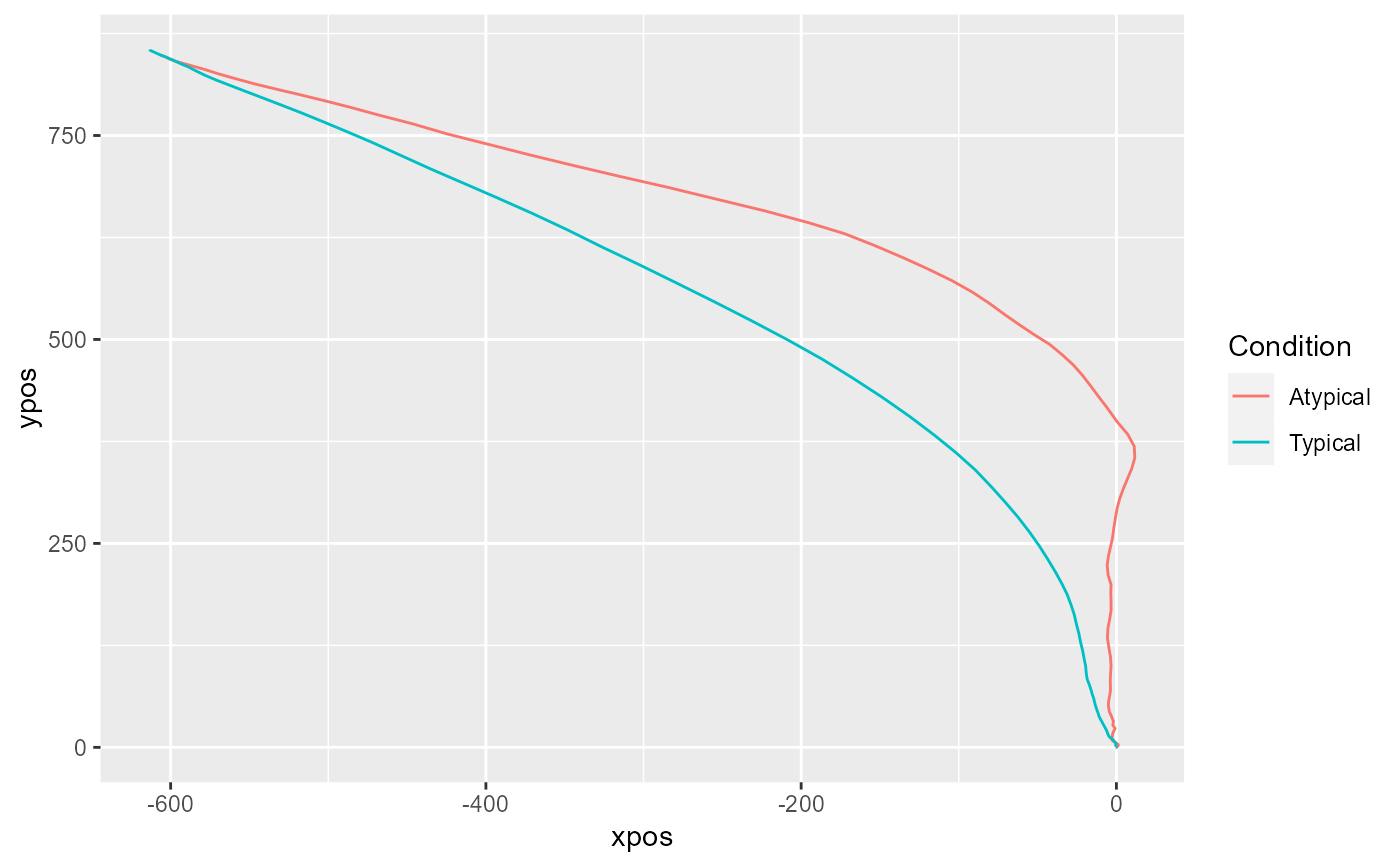The mousetrap package provides functions for importing, preprocessing, analyzing, aggregating, and visualizing mouse-tracking data. In the following, a brief overview of the functions in this package is given.

Depending on the file format, one of the standard R functions for reading files into R can be used (e.g., read.table or read.csv).

If raw data were collected using MouseTracker, the mousetrap package provides the read_mt function to read files in the ".mt" format.

If several raw data files should be read and merged, the read_bulk function from the readbulk package can be used (or the read_opensesame function, if data were collected using OpenSesame).

## Import functions

The initial step to prepare data for analysis in the mousetrap package is to create a mousetrap data object. Depending on the input format, one of the following functions can be used. A detailed description (and example) of the resulting mousetrap data object can be found in mt_example.

mt_import_mousetrap imports mouse-tracking data that were recorded using the mousetrap plugin for OpenSesame.

mt_import_wide imports mouse-tracking data saved in a wide format (e.g., data collected using MouseTracker).

mt_import_long imports mouse-tracking data saved in a long format. (e.g., trajectories exported using mt_export_long).

## Geometric preprocessing functions

A number of functions are available that perform geometric preprocessing operations.

mt_remap_symmetric remaps mouse trajectories to one side (or one quadrant) of the coordinate system.

mt_align is a general purpose function for aligning and rescaling trajectories. For specific operations, you can rely on one of the following functions.

mt_align_start aligns the start position of trajectories.

mt_align_start_end aligns all trajectories so that they share a common initial and final coordinate (this is also sometimes referred to as "space-normalization").

## Resampling and interpolation functions

A number of functions are available that perform resampling and interpolation operations.

mt_exclude_initiation excludes the initial phase of a trial without mouse movement.

mt_time_normalize performs time-normalization using equidistant time intervals, resulting in an identical number of samples for all trajectories.

mt_resample resamples trajectories so that samples occur at constant intervals of a specified length.

mt_average averages trajectory coordinates (and related variables) for time bins of constant duration.

mt_spatialize re-represents each trajectory spatially so that adjacent points on the trajectory become equidistant to each other.

## Data handling functions

A number of functions are available for data handling operations, such as filtering or adding of new variables or trajectories.

mt_subset filters mouse-tracking data by trials, so that only those meeting the defined criteria are included.

mt_bind joins two trajectory arrays.

## Analysis functions

A number of different analysis procedures and summary statistics for mouse trajectories have been established in the existing literature. The following functions implement many of these approaches.

mt_derivatives calculates distance, velocity, and acceleration for trajectories.

mt_angles calculates movement angles for trajectories.

mt_deviations calculates the deviations from an idealized trajectory (straight line).

mt_measures calculates a set of mouse-tracking measures.

mt_sample_entropy calculates sample entropy.

mt_standardize standardizes mouse-tracking measures onto a common scale (separately for subsets of the data, e.g., per participant).

mt_scale_trajectories provides different options for standardizing variables in a mouse trajectory array.

mt_check_bimodality assesses the bimodality of mouse-tracking measure distributions.

mt_check_resolution checks the (temporal) logging resolution of raw trajectories.

mt_count counts the number of observations for each trajectory.

## Cluster functions

A number of different functions for clustering trajectories is provided.

mt_distmat computes the dissimilarity/distance between each pair of trajectories.

mt_cluster performs trajectory clustering with a specified number of clusters.

mt_cluster_k estimates the optimal number of clusters using various methods.

mt_map maps trajectories onto a predefined set of prototype trajectories (a core set is provided in mt_prototypes).

## Reshaping, aggregation, and export functions

A number of helper functions are provided for aggregating, plotting, and exporting the multi-dimensional mouse trajectory arrays.

mt_reshape is a general purpose reshaping and aggregation function for mousetrap data.

mt_aggregate aggregates mouse-tracking data per condition.

mt_aggregate_per_subject aggregates mouse-tracking data per (within subjects-) condition separately for each subject.

mt_export_long exports mouse-tracking data in long format.

mt_export_wide exports mouse-tracking data in wide format.

## Visualization functions

The following functions can be used for plotting trajectory data, e.g., individual and aggregated trajectories or velocity profiles.

mt_plot plots individual trajectory data.

mt_plot_aggregate plots aggregated trajectory data.

mt_plot_riverbed plots the relative frequency of a selected variable across time.

mt_plot_per_trajectory creates a pdf with separate plots per trajectory.

mt_heatmap and mt_heatmap_ggplot plot trajectory heatmaps.

mt_diffmap for creating a difference-heatmap of two trajectory heatmap images.

mt_animate creates a gif trajectory animation.

## Helper functions

bimodality_coefficient calculates the bimodality coefficient.

scale_within scales and centers variables within the levels of another variable.

bezier creates Bezier-curves using the Bernstein approximation.

## Datasets

mt_example and mt_example_raw contain a mouse-tracking example dataset for demonstrations using the mousetrap package.

KH2017 and KH2017_raw contain a mouse-tracking dataset from Kieslich & Henninger (2017).

## Examples

if (FALSE) {
KH2017 <- mt_import_mousetrap(subset(KH2017_raw,correct==1))
KH2017 <- mt_remap_symmetric(KH2017)
KH2017 <- mt_align_start(KH2017)
}

KH2017 <- mt_time_normalize(KH2017)
KH2017 <- mt_measures(KH2017)

mt_aggregate(
KH2017, use="measures",
use2_variables="Condition",
subject_id="subject_nr"
)}